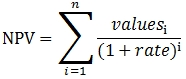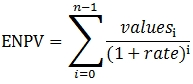# SQL Server 2005 net present value function

NPV_q

Updated: 5 August 2010

Use NPV_q to calculate the net present value of an investment based on a series of periodic cash flows and a discount rate.

If n is the number of cash flows in the list of values, the formula for NPV is:Whereas the formula for ENPV is:Where
N = Number of cashflows
Values = Cashflow amounts
Rate = Discount rate
Syntax
SELECT [westclintech].[wct].[NPV_q] (
<@Rate, float,>
,<@Cashflows_RangeQuery, nvarchar(4000),>)
Arguments
@Rate
The rate to be used for discounting the cash flows in calculating the net present value. @Rate is an expression of type float or of a type that can be implicitly converted to float.
@Cashflows_RangeQuery
a select statement, as text, which specifies the cash flow values to be used in the net present value calculation.
Return Type
float
Remarks
·         For the results to be meaningful, the cash flows should be equally spaced with respect to time and occur at the end of each period.
·         Order matters, so make sure that your SELECT statement uses a meaningful index or specify the order of the cash flows explicitly in the WHERE clause.
·         NPV is related to the IRR function in that IRR is the value which when used to discount the cash flows returns an NPV of zero.
·         It is important to be consistent with the units for @Rate and @Nper. For example if payments are to be paid monthly, then @Rate should be the monthly rate, which can be specified as the annual rate divided by 12. If payments are made quarterly, divide the annual rate by 4. If payments are made semi-annually, divide the annual rate by 2.
·         Funds that are paid should be represented with negative numbers. Funds that are received should be represented as positive numbers.
·         NPV_q returns results consistent with the EXCEL NPV function, which assumes that the first cash flow is discounted at the discount rate; in most financial texts the first cash flow is not discounted. Use the ENPV_q function for that treatment. The ENPV_q result divided by the NPV result should be equal to 1 plus the discount rate
Example

Create a table to store cash flow projections, by year, for a variety of projects:
CREATE TABLE [dbo].[cf1](
[proj_no] [float] NOT NULL,
[period] [float] NOT NULL,
[cf_amt] [float] NOT NULL,
CONSTRAINT [PK_cf1] PRIMARY KEY CLUSTERED
(
[proj_no] ASC,
[period] ASC
)WITH (PAD_INDEX = OFF, STATISTICS_NORECOMPUTE = OFF, IGNORE_DUP_KEY = OFF, ALLOW_ROW_LOCKS = ON, ALLOW_PAGE_LOCKS = ON) ON [PRIMARY]
) ON [PRIMARY]
Insert the data for 3 projects into the table.
INSERT INTO cf1 VALUES(1,1,-25000)
INSERT INTO cf1 VALUES(1,2,5000)
INSERT INTO cf1 VALUES(1,3,10000)
INSERT INTO cf1 VALUES(1,4,15000)
INSERT INTO cf1 VALUES(1,5,20000)
INSERT INTO cf1 VALUES(1,6,25000)
INSERT INTO cf1 VALUES(2,1,-25000)
INSERT INTO cf1 VALUES(2,2,25000)
INSERT INTO cf1 VALUES(2,3,10000)
INSERT INTO cf1 VALUES(2,4,15000)
INSERT INTO cf1 VALUES(2,5,10000)
INSERT INTO cf1 VALUES(2,6,5000)
INSERT INTO cf1 VALUES(3,1,-25000)
INSERT INTO cf1 VALUES(3,2,5000)
INSERT INTO cf1 VALUES(3,3,25000)
INSERT INTO cf1 VALUES(3,4,10000)
INSERT INTO cf1 VALUES(3,5,20000)
INSERT INTO cf1 VALUES(3,6,15000)

Enter a SELECT statement to calculate the NPV for the 3 projects so as to compare the results, assuming a 10% rate:
select d.proj_no
,wct.NPV_q(.10
,'SELECT c.cf_amt
from cf1 c
where c.proj_no = ' + convert(char, d.proj_no)) as NPV
from cf1 d
group by d.proj_no

Here is the result set
proj_no                NPV
---------------------- ----------------------
1                      25693.5832297053
2                      24723.8170178729
3                      27903.4986658659

### SupportCopyright 2008-2021 Westclintech LLC         Privacy Policy        Terms of Service